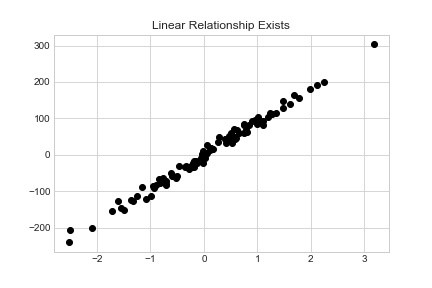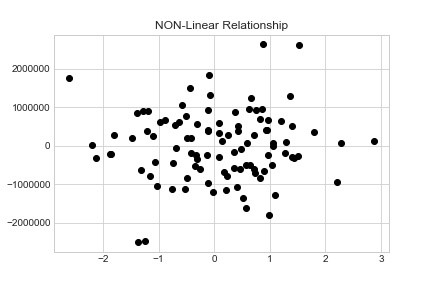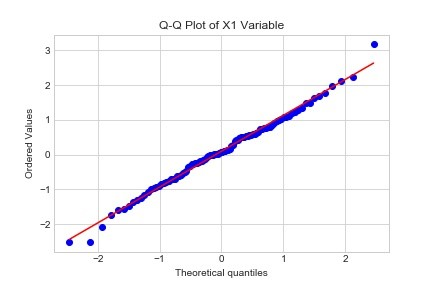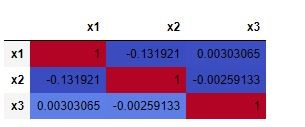Assumptions of Linear Regression with Python

Linear regression is a well known predictive technique that aims at describing a linear relationship between independent variables and a dependent variable. Regression analysis is probably amongst the very first you learn when studying predictive algorithms. As simple as it seems (once you have used it enough), it is still a powerful technique widely used in statistics and data science. In this article, we will go through the assumptions you must test your data for in order to correctly apply linear regression.

Linear regression analysis has five key assumptions. These are:

• We are investigating a linear relationship
• All variables follow a normal distribution
• There is very little or no multicollinearity
• There is little or no autocorrelation
• Data is homoscedastic

Investigating a Linear Relationship

Linear in the name says it all, we are aiming at finding a linear relationship between the independent and dependent variables when running a linear regression model. A simple visual way of determining this is through the use of scatter plots.

In this exercise we will use sklearn to generate our dataset using the make_regression function and then utilize matplotlib to quickly generate our scatterplots to visualize inspect if a linear relationship exists.

Import the required packages.

%matplotlib inline
import matplotlib.pyplot as plt
plt.style.use('seaborn-whitegrid')

from sklearn.datasets.samples_generator import make_regression

Next, we generate a dataset using the make_regression function of sklearn. We will keep the noise parameter low so that our dataset does follow a linear relationship. The noise parameter defines the standard deviation present in our dataset. Finally, plot the x1 and y1 variables using matplotlib’s plot function.

from sklearn.datasets.samples_generator import make_regression
x1, y1 = make_regression(n_samples=100, n_features=1, noise=10)

plt.plot(x1, y1, 'o', color='black');
plt.title("Linear Relationship Exists")As you can see from the image above, there is a linear relationship between the x1 and y1 variable.

Now, let’s take a look at how a nonlinear relationship would look like. We follow the sames steps as above but instead make the noise parameter a much larger number, in my case I will use 900000.

x2, y2 = make_regression(n_samples=100, n_features=1, noise=900000)

plt.plot(x2, y2, 'o', color='black');
plt.title("NON-Linear Relationship")Clearly from the scatter plot you can quickly tell there is no linear relationship between the x2 and y2 variables. In this case, running a linear regression model won’t be of help.

Variables follow a Normal Distribution

The next assumption is that the variables follow a normal distribution. In order words we want to make sure that for each x value, y is a random variable following a normal distribution and its mean lies on the regression line. To take a deeper dive into probability distributions with python you can read this article: Fitting Probability Distributions with Python

One of the ways to visually test for this assumption is through the use of the Q-Q-Plot. Q-Q stands for Quantile-Quantile plot and is a technique to compare two probability distributions in a visual manner.

To generate this Q-Q plot we will be using scipy’s probplot function where we compare a variable of our chosen to a normal probability.

import scipy.stats as stats

stats.probplot(x1[:,0], dist="norm", plot=plt)
plt.show()How do you know if your variable follows a normal distribution? You see the red line in the chart above? The points must lie on this line to conclude that it follows a normal distribution. In our case, yes it does! The couple of points outside of the line is due to our small sample size. In practice, you decide how strict you want to be as it is a visual test.

There is little or no Multicollinearity

Multicollinearity is a fancy way of saying that your independent variables are highly correlated with each other. Remember the name of your X’s, they are called independent variables for a reason. If multicollinearity exists between them, they are no longer independent and this generates issues when modeling linear regressions.

To visually test for multicollinearity we can use the power of Pandas and their styling options (in development) which allows us to style data frames according to the data within them.

First, let’s create a regression dataset as we did in the first example, but this time having it return 3 X variables. We then convert this array into a pandas dataframe and use the inbuilt Pandas corr function to compute the pairwise correlation of our columns.

#create sample dataset with 3 x features
x3, y3 = make_regression(n_samples=100, n_features=3, noise=20)

#convert to a pandas dataframe
import pandas as pd
df = pd.DataFrame(x3)
df.columns = ['x1','x2','x3']

#generate correlation matrix
corr = df.corr()

With our corr variable holding the correlation matrix, apply styling to using the coolwarm color map. Low values will have a blue color while higher values will become “hot” and thus the red.If you find any values which the absolute value of their correlation is >=0.8, the multicollinearity assumption is being broken.

There is little or no AutoCorrelation

This next assumption ir much like our previous one, except it applies to the residuals of your linear regression model. Because creating a linear regression model is outside the scope of this article, we won’t go deeper into this assumption until our next article when we delve into running a linear regression model.

Homoscedasticity

The last assumption of linear regression is that of homoscedasticity, this analysis is also applied to the residuals of your linear regression model and can be easily tested with a scatterplot of the residuals.

Homoscedasticity is present when the noise of your model can be described as random and same throughout all independent variables. If by looking at the scatterplot of the residuals from your linear regression analysis you notice a pattern, this is a clear sign that this assumption is being violated.

Conclusion

In this article we used python to test the 5 key assumptions of a linear regression. The first three are applied before you begin a regression analysis, while the last 2 (AutoCorrelation and Homoscedasticity) are applied to the residual values once you have completed the regression analysis. You are now armed with the knowledge to decide if linear regression is the right model to utilize for your specific use case. In upcoming articles, we will run through a linear regression model in python.